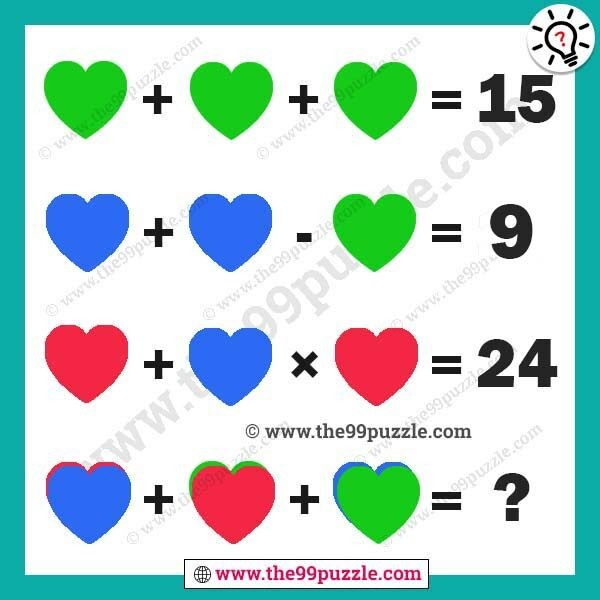# Love picture logical math equation for genius – Puzz173

Love picture logical math equation for genius. Math logic picture puzzles for genius minds. These picture puzzles are challenging for people. If you are genius people you can calculate the math equation picture puzzle.###### Explanation:

Green heart + Green heart + Green heart = 15

Blue heart + Blue heart – Green heart = 9

Red heart + Blue heart × Red heart = 24

(Red heart+Blue heart) + (Green heart+Red heart) + (Blue heart+Green heart) = ?

5 + 5 + 5 = 15

7 + 7 – 5 = 9

3 + 7 × 3 = 24

(3+7) + (5+3) + (7+5) = 30